# Preface

This part of tutorial demonstrates tremendous plotting capabilities of Mathematica for three-dimensional figures. Plain plotting was given in the first part of tutorial. Of course, we cannot present all features of Mathematica's plotting in one section, so we emphasize some important techniques useful for creating figures in three dimensions. Other graphs are demonstrated within tutorial when visualization is needed.

Introduction to Linear Algebra with Mathematica

# Introduction: 3D Plotting

Example 1: The paraboloid example shows that any function with three variables can be visually represented using the Plot3D function. Also, the ColorFunction option allows you to easily see the changes of the function.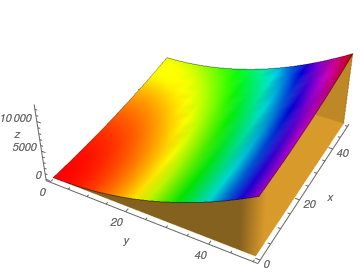Paraboloid. Plot3D[4*x^2 + y^2, {x, 0, 50}, {y, 0, 50}, Axes -> True, AxesLabel -> {y, x, z}, Boxed -> False, LabelStyle -> Italic, Mesh -> False, Filling -> Bottom, FillingStyle -> Opacity[.8], ColorFunction -> Hue, AspectRatio -> Automatic]
■

Example 2: The VectorPlot3D function is used to plot vector fields. Vector fields are used to represent equations that model many physical quantities such as the flow of liquid, strength of a force, and velocity of an object.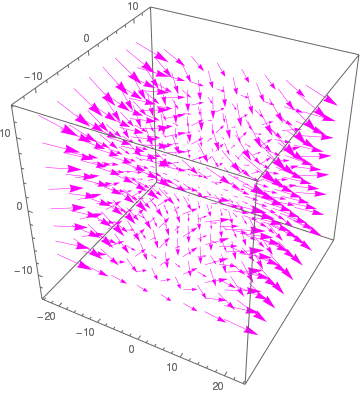Vector plot. VectorPlot3D[{2*x^2, 3*y^2, -4*z^2}, {x, -20, 20}, {y, -10, 10}, {z, -10, 10}, BoxRatios -> {1, 1, 1}, VectorStyle -> Magenta]
■
Example 3: Viviani's curve is called after the Italian mathematician and scientist Vincenzo Viviani (1622--1703). He was a pupil of Torricelli and a biographer of Galileo, who studied the curve in 1692) is the intersection of the cylinder $$(x-a)^2 +y^2 =a^2$$ and the sphere $$x^2 + y^2 + z^2 = 4a^2$$. It is parametrized by

$$\mbox{viviani}[a](t) = a (1+\cos t , \sin t, 2\sin (t/2)) \qquad (-2\pi \le t \le 2\pi )$$

To plot the viviani curve, we first define its equation and then plot it

viviani[a_][t_] := a {1+ Cos[t], Sin[t], 2*Sin[t/2]}
ParametricPlot3D[viviani[1.2][t], {t, 0, 13}, PlotStyle -> Thick]
The three dimensional picture is obtained with the following code.
a = ParametricPlot3D[{1 + Sin[t], Cos[t], u}, {t, 0, 2*Pi}, {u, -2, 2}, Mesh -> Full, PlotStyle -> Blue]
b = ParametricPlot3D[{2*Cos[t]*Cos[u], 2*Sin[t]*Cos[u], 2*Sin[u]}, {t, 0, 2*Pi}, {u, -Pi, Pi}, PlotStyle -> LightRed]
Show[b, a]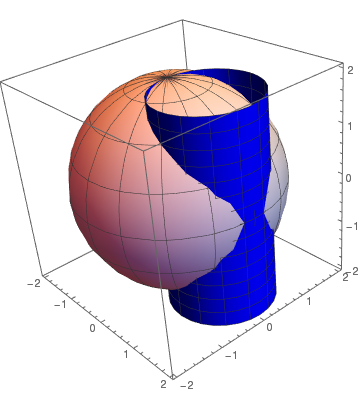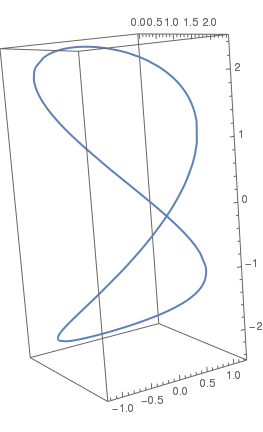Vincenzo Viviani Intersection of a cylinder with sphere Viviani curve
Here is another variation of the viviani curve:
ParametricPlot3D[{Cos[t] Sin[t], (Sin[t])^2 , Cos[t]}, {t, 0, 2 Pi}]
■
Example 4: Some examples of three dimensional regions.
RegionPlot3D[ x^2 + y^2 <= 36 && y <= (1/Sqrt)*x, {x, 0, 6}, {y, 0, 6}, {z, 0, 3}, BoxRatios -> Automatic, AxesLabel -> Automatic]

or
R = RegionProduct[ DiscretizeRegion[Disk[{0, 0}, 1, {0, Pi/8}], MaxCellMeasure -> 0.01], Line[{{0.}, {0.5}}]] RegionPlot3D[R]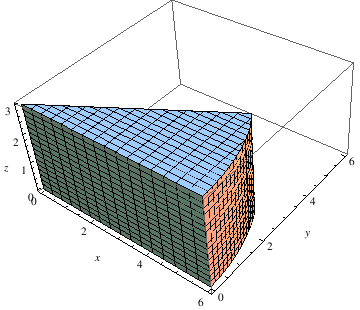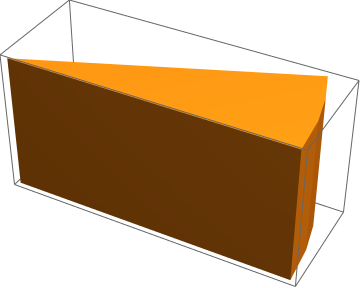Now we randomly cut out some balls, using the RandomPoint function, to obtain a piece of cheese. This code takes a while to generate an output due to the randomness. However, each time you run the code, a new figre will appear. The following code creates a random number of balls of radius 0.65 within the three dimensional region.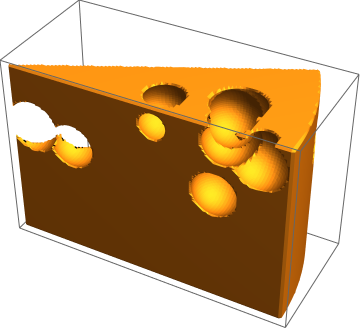Cheese. RegionPlot3D[ Fold[RegionDifference, ImplicitRegion[ x^2 + y^2 <= 6^2 && 0 < z < 4 && 0 < y <= x Sin[Pi/6], {x, y, z}], Ball[#, 0.65] & /@ RandomPoint[ ImplicitRegion[ x^2 + y^2 <= 6^2 && 0 < z < 4 && 0 < y <= x Sin[Pi/6], {x, y, z}], 10]], PlotPoints -> 100]

The following code creates a random number of balls of radius 0.35 within the three dimensional region.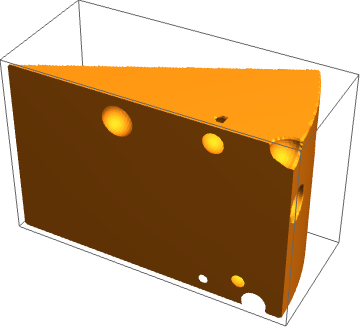Cheese with balls of upper fixed size RegionPlot3D[ Fold[RegionDifference, ImplicitRegion[ x^2 + y^2 <= 6^2 && 0 <= z <= 4 && 0 <= y <= x Sin[Pi/6], {x, y, z}], Ball[#, 0.35] & /@ RandomPoint[ImplicitRegion[x^2 + y^2 <= 6^2 && 0 < z < 4 && 0 < y <= x Sin[Pi/6], {x, y, z}], 10]], PlotPoints -> 100]

The following code show more examples of plotting three dimensional regions. You may want to move the figure around to see the entire shape!Pack. RegionPlot3D[x^2 + y^2 <= 16, {x, -4, 4}, {y, -4, 4}, {z, 0, 2}, BoxRatios -> Automatic, AxesLabel -> Automatic, PlotStyle -> Gray, Mesh -> 8]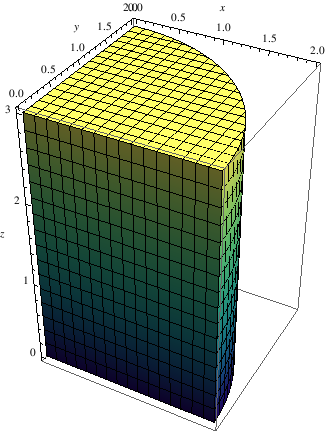Quarter of pack. RegionPlot3D[x^2 + y^2 <= 4, {x, 0, 2}, {y, 0, 2}, {z, 0, 3}, BoxRatios -> Automatic, AxesLabel -> Automatic, ColorFunction -> "BlueGreenYellow"]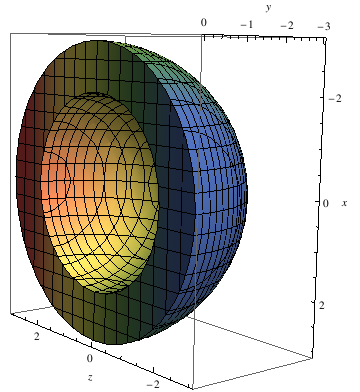Bowl. RegionPlot3D[ x^2 + y^2 + z^2 <= 9 && x^2 + y^2 + z^2 >= 4, {x, -3, 3}, {y, -3, 0}, {z, -3, 3}, BoxRatios -> Automatic, AxesLabel -> Automatic, ColorFunction -> "DarkRainbow", MeshShading -> Automatic]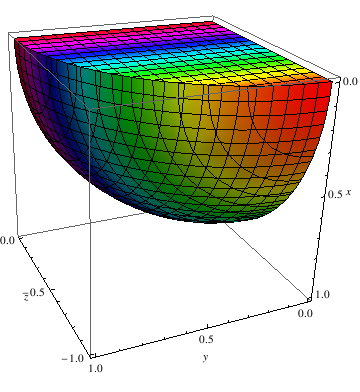Eighth part of the ball. RegionPlot3D[x^2 + y^2 + z^2 <= 1, {x, 0, 1}, {y, 0, 1}, {z, -1, 0}, BoxRatios -> Automatic, AxesLabel -> Automatic, ColorFunction -> Hue, MeshShading -> Automatic]
■

Example 5: Logarithmic functions are used to represent real world situations like population growth, decay of a substance, and financial interest rates. Most times these functions are shown in 2 dimensional spaces, but can still be represented in 3D.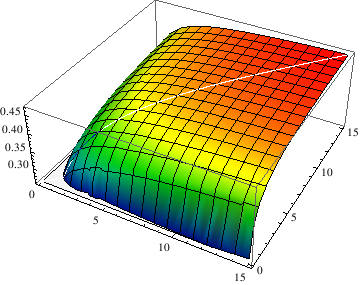Logarithmic function. G[x_, y_] := (Log[ Min[1, (1 + x)/(1 + y)]* Min[1, (1 + y)/(1 + x)]])/(Log[(Min[x/y, y/x])^2]) Plot3D[G[x, y], {x, 0.1, 15}, {y, 0.1, 15}, ColorFunction -> Function[{x, y, z}, Hue[0.65*(1 - z)]]]
■

Example 6: Now we plot surface of revolution with respect to x-axis. Using the RevolutionPlot3D function, you can create a simple 2D function into a cool 3D shape:

RevolutionPlot3D[{t^2, t}, {t, 0, 4}, {$Theta], 0, 2 Pi}, RevolutionAxis -> "X"] RevolutionPlot3D[{Sin[t], t}, {t, 0, 2 Pi}, {\[Theta], 0, Pi}, ColorFunction -> "Rainbow"]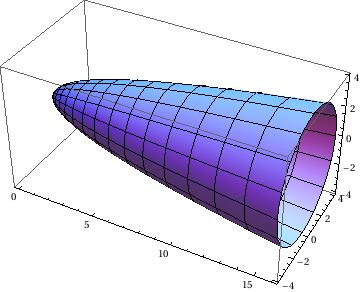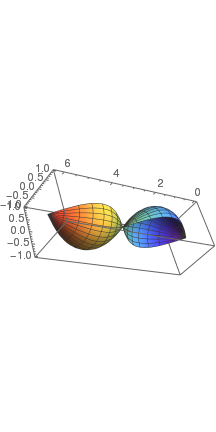Revolution of parabola Revolution of sine Now we plot a fancy ambrella: RevolutionPlot3D[Exp[-x], {x, 0, 2}, ColorFunction -> "NeonColors"]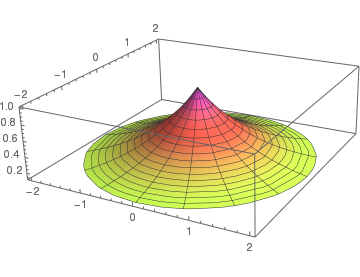Example 7: A catenoid is a type of surface, arising by rotating a catenary curve about an axis. It is a minimal surface, meaning that it occupies the least area when bounded by a closed space. It was formally described in 1744 by the mathematician Leonhard Euler. The catenoid may be defined by the following parametric equations: \[ \begin{split} x(u,v) &= c\,\cosh \left( \frac{v}{c} \right) \cos u , \\ y(u,v) &= c\,\cosh \left( \frac{v}{c} \right) \sin u , \\ z(v) &= v , \end{split}$
where u ∈ [-π,π) and v ∈ ℝ and c is a non-zero real constant.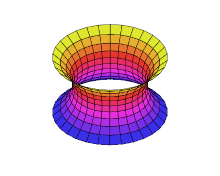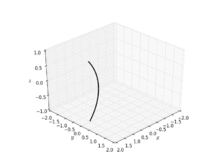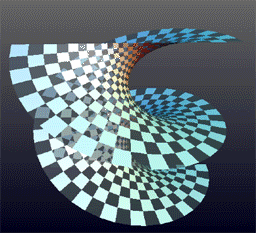A helicoid minimal surface formed by a soap film on a helical frame A catenoid A catenoid obtained from the rotation of a catenary Deformation of a helicoid into a catenoid

Example 8:

a = SphericalPlot3D[{1}, {\[Theta], 0, 2 Pi}, {\[Phi], 0, 4 Pi/2}, PlotStyle -> Directive[Blue, Opacity[0.5], Specularity[White, 20]], Mesh -> None, PlotPoints -> 450];
b = SphericalPlot3D[{2}, {\[Theta], 0, Pi}, {\[Phi], 0, 5 Pi/3}, PlotStyle -> Directive[Blue, Opacity[0.5], Specularity[White, 20]], Mesh -> {{0}, {0}, {0}}, PlotPoints -> 50];
c = Graphics3D[Arrow[Tube[{{2, 2, 2}, {1, 0, 0}}, .03]]];
d = Graphics3D[Arrow[Tube[{{0, -3, 1}, {0, -2, 1}}, .03]]];
e = Graphics3D[Arrow[{{0, 0, 0}, {3, 0, 0}}]];
f = Graphics3D[Arrow[{{0, 0, 0}, {0, -3, 0}}]];
g = Graphics3D[Arrow[{{0, 0, 0}, {0, 0, 3}}]];
h = Graphics3D[Arrow[{{0, 0, 0}, {-3, 0, 0}}]];
i = Graphics3D[Arrow[{{0, 0, 0}, {0, 3, 0}}]];
j = Graphics3D[Arrow[{{0, 0, 0}, {0, 0, -3}}]];
k = Graphics3D[Text[P == 1, {2, 2, 2}]];
l = Graphics3D[Text[P == 2, {0, -3, 1}]];
m = Graphics3D[Text[x, {0, -3.1, 0}]];
n = Graphics3D[Text[y, {3.1, 0, 0}]];
o = Graphics3D[Text[z, {0, 0, 3}]];
az = Show[a, b, c, d, e, f, g, h, i, j, k, l, m, n, o, PlotRange -> {{-3, 3}, {-3, 3}, {-3, 3}}, Axes -> {False, False, False}, Boxed -> False] We can plot sphere with different colors.

a = SphericalPlot3D[{1}, {\[Theta], 0, 2 Pi}, {\[Phi], 0, 4 Pi/2}, PlotStyle -> Directive[Red, Opacity[0.5], Specularity[White, 20]], Mesh -> None, PlotPoints -> 450];
b = SphericalPlot3D[{2}, {\[Theta], 0, Pi}, {\[Phi], 0, 5 Pi/3}, PlotStyle -> Directive[Blue, Opacity[0.5], Specularity[White, 20]], Mesh -> {{0}, {0}, {0}}, PlotPoints -> 50];
c = Graphics3D[Arrow[Tube[{{2, 2, 2}, {1, 0, 0}}, .03]]];
d = Graphics3D[Arrow[Tube[{{0, -3, 1}, {0, -2, 1}}, .03]]];
e = Graphics3D[Arrow[{{0, 0, 0}, {3, 0, 0}}]];
f = Graphics3D[Arrow[{{0, 0, 0}, {0, -3, 0}}]];
g = Graphics3D[Arrow[{{0, 0, 0}, {0, 0, 3}}]];
h = Graphics3D[Arrow[{{0, 0, 0}, {-3, 0, 0}}]];
i = Graphics3D[Arrow[{{0, 0, 0}, {0, 3, 0}}]];
j = Graphics3D[Arrow[{{0, 0, 0}, {0, 0, -3}}]];
k = Graphics3D[Text[P == 1, {2, 2, 2}]];
l = Graphics3D[Text[P == 2, {0, -3, 1}]];
m = Graphics3D[Text[x, {0, -3.1, 0}]];
n = Graphics3D[Text[y, {3.1, 0, 0}]];
o = Graphics3D[Text[z, {0, 0, 3}]];
az = Show[a, b, c, d, e, f, g, h, i, j, k, l, m, n, o, PlotRange -> {{-3, 3}, {-3, 3}, {-3, 3}}, Axes -> {False, False, False}, Boxed -> False]Two spheres Two spheres with different colors

If you don't need any arrows and text, it can be plotted easier:

c = SphericalPlot3D[4, {\[Theta], 0, \[Pi]}, {\[Phi], 1, 2 \[Pi]}]
d = SphericalPlot3D[2, {\[Theta], 0, Pi}, {\[Phi], 0, 2 Pi}]
Show[c, d]

Another plot with different colors:
c = SphericalPlot3D[4, {\[Theta], 0, \[Pi]}, {\[Phi], 1, 2 \[Pi]}, Mesh -> None, PlotStyle -> {Opacity[0.6], Purple}];
d = SphericalPlot3D[2, {\[Theta], 0, Pi}, {\[Phi], 0, 2 Pi}, Mesh -> None, PlotStyle -> Yellow];
Show[c, d]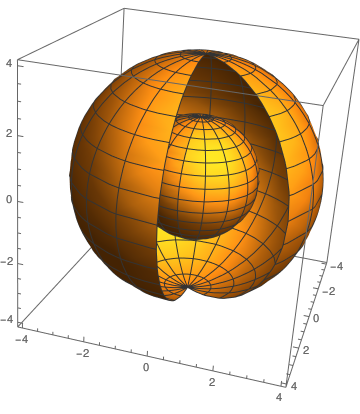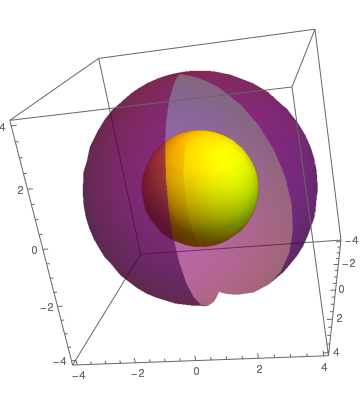Two spheres Two spheres with different colors

The following script can be used to display arrows and text to specifically illustrate dimensions.

a = Graphics3D[Arrow[Tube[{{0, 0, 1}, {0, 0, 2}}, .025]]];
c = Graphics3D[Arrow[Tube[{{0, 0, 1}, {0, 0, 0}}, .025]]];
o = Graphics3D[Text[height, {-0.5, -0.5, 1}]];
p = Graphics3D[Arrow[Tube[{{1, 0, 1}, {0, 0, 1}}, .025]]];
q = Graphics3D[Arrow[Tube[{{1, 0, 1}, {1.75, 0, 1}}, .025]]];
r = Graphics3D[Text[radius, {1, 0, 0.8}]];
d = Graphics3D[Cylinder[{{0, 0, 0}, {0, 0, .0001}}, 2]];
b = SphericalPlot3D[{2}, {\[Theta], 0, Pi}, {\[Phi], 0, 3 Pi/2}, PlotStyle -> Directive[Red, Opacity[0.7], Specularity[White, 20]], Mesh -> {{0}, {0}, {0}}, PlotPoints -> 40];
g = Graphics3D[Arrow[{{0, 0, 0}, {0, 0, 3}}]];
h = Graphics3D[Arrow[{{0, 0, 0}, {0, -3, 0}}]];
i = Graphics3D[Arrow[{{0, 0, 0}, {3, 0, 0}}]];
j = Graphics3D[Text[z, {0, 0, 3.2}]];
k = Graphics3D[Text[x, {3.2, 0, 0}]];
l = Graphics3D[Text[y, {0, -3.2, 0}]];
m = Graphics3D[Arrow[{{0, 0, 0}, {-3, 0, 0}}]];
n = Graphics3D[Arrow[{{0, 0, 0}, {0, 3, 0}}]];
b = Show[a, b, c, d, g, h, i, j, k, l, m, n, o, p, q, r, PlotRange -> {{-4, 4}, {-4, 4}, {0, 4}}]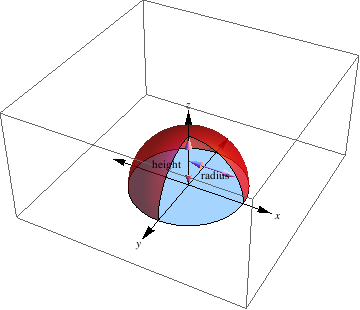Without showing dimensions:

a = Graphics3D[Arrow[Tube[{{0, 0, 0}, {0, 0, Sqrt}}, .025]]];
c = Graphics3D[Arrow[Tube[{{0, 0, 0}, {1, 0, 1}}, .025]]];
d = Graphics3D[Cylinder[{{0, 0, 0}, {0, 0, .0001}}, 2]];
b = SphericalPlot3D[{2}, {\[Theta], 0, Pi}, {\[Phi], 0, 5 Pi/4}, PlotStyle -> Directive[Red, Opacity[0.7], Specularity[White, 20]], Mesh -> {{0}, {0}, {0}}, PlotPoints -> 40];
g = Graphics3D[Arrow[{{0, 0, 0}, {0, 0, 3}}]];
h = Graphics3D[Arrow[{{0, 0, 0}, {0, -3, 0}}]]; i = Graphics3D[Arrow[{{0, 0, 0}, {3, 0, 0}}]];
j = Graphics3D[Text[z, {0, 0, 3.2}]];
k = Graphics3D[Text[y, {3.2, 0, 0}]];
l = Graphics3D[Text[x, {0, -3.2, 0}]];
m = Graphics3D[Arrow[{{0, 0, 0}, {-3, 0, 0}}]];
n = Graphics3D[Arrow[{{0, 0, 0}, {0, 3, 0}}]];
b = Show[a, b, c, d, g, h, i, j, k, l, m, n, PlotRange -> {{-4, 4}, {-4, 4}, {0, 4}}]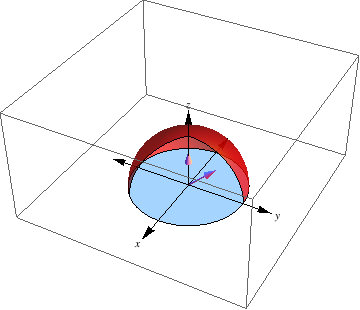The following code demonstrate how you can make shapes interact with each other. Multiple images may appear because the first shapes overlap to create the final shape needed. Don't forget about being able to rotate the shape! You can cut a piece of a sphere:

RegionPlot3D[ x^2 + y^2 <= 6^2 && 0 < z < 4 && 0 < y <= .1 x Sin[Pi/6], {x, 0, 6}, {y, 0, Sin[Pi/6]}, {z, 0, 4}]
a = SphericalPlot3D[4, {\[Theta], 0, \[Pi]/2}, {\[Phi], 1, 2 \[Pi]}]
b = SphericalPlot3D[4, {\[Theta], .8, \[Pi]/2}, {\[Phi], 0, 2 \[Pi]}]
Show[a, b]One can put a box inside a sphere:

e = SphericalPlot3D[4, {\[Theta], 0, \[Pi]/2}, {\[Phi], 3, 2 \[Pi]}]
f = Graphics3D[Cuboid[{-2, -1, 2}]]
Show[e, f]

e = SphericalPlot3D[4, {\[Theta], 0, \[Pi]/2}, {\[Phi], 3, 2 \[Pi]}, MeshShading -> {{White, Black}, {Blue, Pink}}] f = Graphics3D[Cuboid[{-2, -1, 2}]] Show[e, f]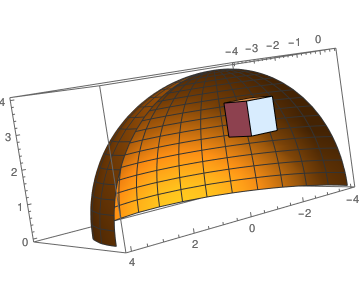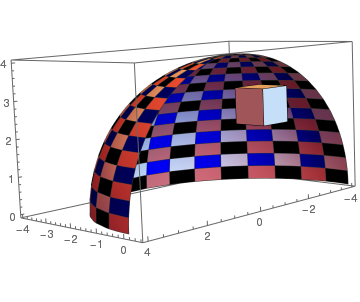Spherical shell with a box Meshed spherical shell with a box

Example 9: Arrow plots can be used to show the direction of a line by adding arrows to the functions. A typical arrow plot:

Arrow[Tube[ Table[{Cos[phi], Sin[phi], .2 phi}, {phi, 0, 10, 0.1}]]] // Graphics3D

or using colors
Graphics3D[{Red, Thickness[Large], Arrow[Tube[ Table[{Cos[phi], Sin[phi], .2 phi}, {phi, 0, 10, 0.1}]]]}]
Line arrow:
Graphics3D[{Cyan, Arrowheads[0.1], Arrow[Tube[{{1, 1, -1}, {2, 2, 0}, {3, 3, -1}, {4, 4, 0}}, 0.05]]}]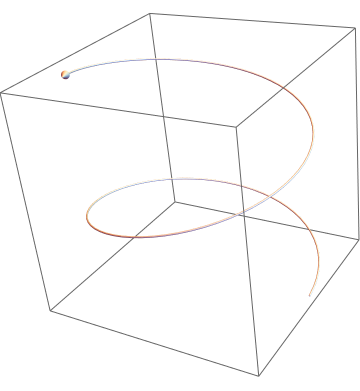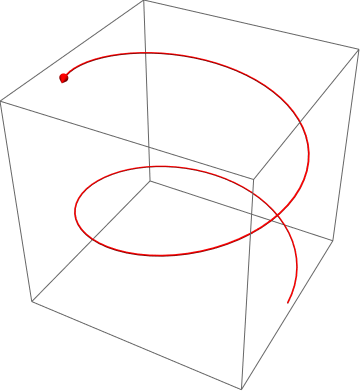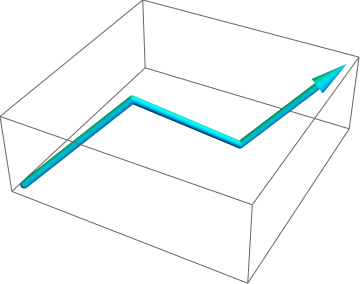Typical arrow Colored arrow Colored line arrow
Example 10: Suppose you need to plot a curve on a given plane with the starting point of the curve on a certain point on the plane. As an example, consider a spiral curve {x[t], y[t]} = {t Cos[2 t], t Sin[2 t]} and a plane: 3 (x + 1) - 2 (y - 2) + (z - 3) = 0. The spiral must be centered at {-1, 2, 3}. Since the given plane is already in a convenient form, we can easily extract the center point and the normal of the plane to use with RotationTransform[] and InfinitePlane[]. Thus,3D spiral With[{pt = {-1, 2, 3}, nrm = {3, -2, 1}}, Show[ParametricPlot3D[ pt + RotationTransform[{{0, 0, 1}, nrm}][ {t Cos[2 t], t Sin[2 t], 0}] // Evaluate, {t, 0, 2 π}], Graphics3D[{{Red, AbsolutePointSize, Point[pt]}, {LightBlue, InfinitePlane[pt, Rest[Orthogonalize[{nrm, {1, 0, 0}, {0, 1, 0}}]]]}}], Lighting -> "Neutral"]]

Or the same plot can be obtained with a sequence of commands. First, we define the point

p = {-1, 2, 3}; point = ListPointPlot3D[{p}, PlotStyle -> {Blue, PointSize[Large]}]

the plane

eqPlane = 3 (x + 1) - 2 (y - 2) + (z - 3) == 0;
f[x_, y_] := z /. First @ Solve[eqPlane, z]
f[x, y]
plane = ParametricPlot3D[{x, y, f[x, y]}, {x, -10, 10}, {y, -10, 10}, Mesh -> None, BoxRatios -> 1

the spiral

{a[t_], b[t_], c[t_]} := {t Cos[2 t], t Sin[2 t], f[a[t], b[t]]}

and the origin of the spiral

s0 = {a, b, c}

Plot of the spiral moved to the point p:

spiral = ParametricPlot3D[{a[t], b[t], c[t]} - s0 + p, {t, 0, 2 π}, PlotStyle -> Red, BoxRatios -> 1]
view = Coefficient[Simplify[First@eqPlane], #] & /@ {x, y, z}
Show[spiral, plane, point, ViewPoint -> view]

Another option is to plot in uninspiring way:

{x[t_], y[t_]} = {t Cos[2 t], t Sin[2 t]};
p = ({x, y, z} /. First@Solve[(x + 1) - 2 (y - 2) + (z - 3) == 0, z])
q = p /. {x -> x[t], y -> y[t]};
Show[Plot3D[Evaluate[p[]], {x, -10, 10}, {y, -10, 10}, Mesh -> None, PlotStyle -> {LightBlue, Opacity[0.5]}, ViewPoint -> {5, 5, 5}], ParametricPlot3D[Evaluate[q], {t, 0, 2 Pi}, PlotStyle -> Red]]

View point (5,5,5) View point (-5,5,5) View point (5,-5,d105)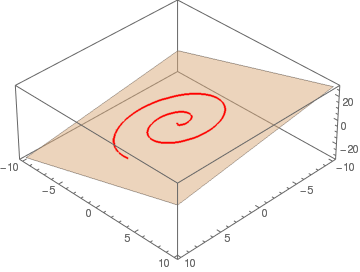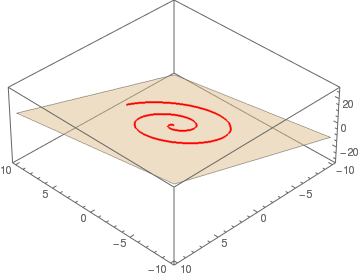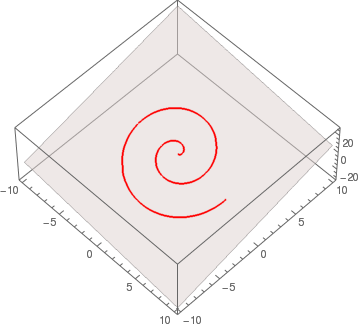Another beautiful command SliceContourPlot3D generates a contour plot of a function over the slice surface as a function of x, y and z. For example, we plot the contours over the surface x³ + 2 y² - 3 z² = 0.Slicing contour plot SliceContourPlot3D[Exp[-(x^2 + y^2 + z^2)], x^3 + 2 y^2 - 3 z^2 == 0, {x,-2,2}, {y,-2,2}, {z,-2,2}]

Ambrella:

RevolutionPlot3D[Exp[-x], {x, 0, 2}]

The function label3D takes an arbitrary expression and displays it as a textured 3D rectangle with transparent background. The expression is converted to an image without being evaluated. By default, regions matching the color at the corner of the image are made transparent. Alternatively, you can explicitly specify which color to make transparent by adding the option "TransparentColor" → White to label3D (instead of White, the color can be anything you like).
label3D[s_, pos_, xVec_, tiltAngle_, opts : OptionsPattern[]] :=
Module[{ra, width, height, r},
ra = Rasterize[
Style[HoldForm[s], FilterRules[{opts}, Options[Style]], Magnification -> 10],
Evaluate@Apply[Sequence, FilterRules[{opts}, Options[Rasterize]]], "Image" ];
{width, height} = ImageDimensions[ra];
r = SetAlphaChannel[ra, With[
{
color = Apply[List, ColorConvert[
"TransparentColor" /. {opts} /. {"TransparentColor" ->
Apply[RGBColor, ImageData[ra][[2, 2]]]}, "RGB"] ]
}, Binarize[ra, (Norm[# - color] > .005)&]]
];
Translate[(* // to make lefthand corner pos *)
Rotate[(* // around z axis *)
Rotate[ (* // around y axis *)
Rotate[(* // tilt around x axis *)
Scale[ (*// to make width equal |xVec| *)
{EdgeForm[FrameStyle /. {opts} /. FrameStyle -> None],
Texture[ImageData@r],
(* // Texture fills polygon initially in the xz plane *)
Polygon[{{0, 0, 0}, {width, 0, 0}, {width, 0, height}, {0, 0, height}},
VertexTextureCoordinates -> {{0, 0}, {1, 0}, {1, 1}, {0, 1}}]},
Norm[xVec]/width, {0, 0, 0} ],
tiltAngle, {1, 0, 0}],(* // x rotation *)
Arg[Chop@N[Norm[xVec[[1 ;; 2]]] + I xVec[]]], {0, -1, 0}], (* // y rotation *)
Arg[Chop@N[xVec[] + I xVec[]]], {0, 0, 1} ], (* // z rotation *)
pos]
];
SetAttributes[label3D, HoldFirst]
The first argument is the object to be displayed. The second argument is the position of the bottom left corner of the label. The third argument is a vector pointing in the direction along which the baseline of the label should be oriented. The length of this vector is taken as the width of the the label. The fourth argument is the angle (in radians) by which the label is rotated around its baseline. The options Magnification (and also ImageSize) determine the resolution of the rasterized label, and also influence the line breaking in wide labels. Without the option FrameStyle, there will be no frame around the label. Here is a simple example showing the relation to the coordinate axes: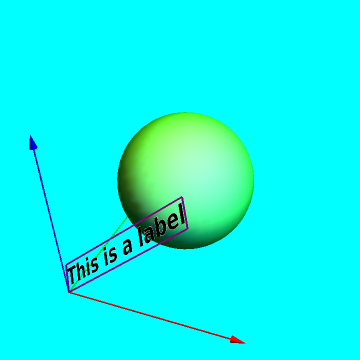Label is included in the graph. To write labels on the graph:     With[{position = {0, 0, 0}, direction = {1.5, 0, 1.3}, tiltAngle = -.8}, Graphics3D[{{Green, Specularity, Sphere[{1, 1, 1}, .7]}, Map[{Apply[RGBColor, #], Arrow[Tube[{{0, 0, 0}, #}]]} &, 2 IdentityMatrix], {Glow[White], label3D["This is a label", position, direction, tiltAngle, FontColor -> Black, FontSize -> 18, FontFamily -> "Helvetica", FontWeight -> Bold, Magnification -> 4, FrameStyle -> Directive[Purple, Thick]]}}, Boxed -> False, SphericalRegion -> True, Background -> Cyan]]

1. Visualization and Graphics by John Boccio, Wolfram Mathematica Tutorial Collection.